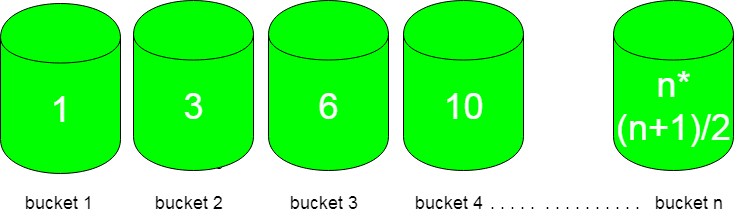# Smallest triangular number larger than p

Given n number of buckets and each bucket is numbered from 1 to n and flowers in it are equal to triangular numbers. You have to choose the bucket which is left with minimum flower after picking ‘p’ flowers from it.First bucket contains only 1 flower, second bucket contains 3, third bucket contains 6 and so on following a pattern of n(n+1)/2.

Examples :

```Input : p = 4
Output : bucket 3
Explanation :
Buckets with flowers : 1 3 6 10 ....
So, bucket 3 is left with only two flowers after
selecting p flowers from it which is minimum.

Input : p = 10
Output : bucket 4
Explanation :
Bucket with flowers : 1 3 6 10 15 ...
So, selecting 10 flowers from 4th bucket leave
it with 0 flowers.
```

## Recommended: Please try your approach on {IDE} first, before moving on to the solution.

Approach :
Observing the input/output of different cases, bucket number can be calculated using formula :

```n = ceil( (sqrt(8*p+1)-1)/2 ) ;
```

How does it work?

We need smallest n such than n*(n+1)/2 >= p

So we need to find roots of equation n2 + n – 2*p >= 0.

By applying the formula discussed here, we get n = ceil( (sqrt(8*p+1)-1)/2 )

## C++

 `// CPP code to find the bucket to choose  ` `// for picking flowers out of it ` `#include ` `using` `namespace` `std; ` ` `  `int` `findBucketNo(``int` `p) ` `{ ` `   ``return` `ceil``( ( ``sqrt``( 8*p + 1 ) -1 ) / 2 ) ; ` `} ` ` `  `// Driver code ` `int` `main() ` `{ ` `  ``int` `p = 10 ;     ` `  ``cout << findBucketNo(p); ` `  ``return` `0; ` `}  `

## Java

 `//Java code to find the bucket to choose  ` `// for picking flowers out of it ` `import` `java.lang.System.*; ` ` `  `class` `GFG { ` `     `  `    ``static` `int` `findBucketNo(``int` `p) ` `    ``{ ` `        ``return` `(``int``)Math.ceil((  ` `        ``Math.sqrt( ``8``*p + ``1` `) -``1` `) / ``2` `) ; ` `    ``} ` `     `  `    ``// Driver code ` `    ``public` `static` `void` `main(String[] args) ` `    ``{ ` `        ``int` `p = ``10` `;  ` `         `  `        ``System.out.println(findBucketNo(p)); ` `    ``} ` `} ` ` `  `// This code is contributed by ` `// Smitha Dinesh Semwal `

## Python 3

 `# Python 3 code to find the bucket  ` `# to choose for picking flowers ` `# out of it ` `import` `math ` ` `  `def` `findBucketNo(p): ` ` `  `    ``return` `math.ceil( ( math.sqrt( ` `               ``8``*``p ``+` `1` `) ``-``1` `) ``/` `2` `)  ` ` `  `# Driver code ` `p ``=` `10` ` `  `print``(findBucketNo(p)) ` ` `  `# This code is contributed by ` `# Smitha Dinesh Semwal `

## C#

 `// C# code to find the bucket to choose  ` `// for picking flowers out of it ` `using` `System; ` ` `  `class` `GFG { ` `     `  `    ``static` `int` `findBucketNo(``int` `p) ` `    ``{ ` `        ``return` `(``int``)Math.Ceiling((  ` `        ``Math.Sqrt( 8*p + 1 ) -1 ) / 2 ); ` `    ``} ` `     `  `    ``// Driver code ` `    ``static` `public` `void` `Main () ` `    ``{ ` `        ``int` `p = 10 ;  ` `        ``Console.WriteLine(findBucketNo(p)); ` `    ``} ` `} ` ` `  `// This code is contributed by Ajit. `

## PHP

 ` `

Output :

```4
```

Time Complexity : O(1)

Attention reader! Don’t stop learning now. Get hold of all the important DSA concepts with the DSA Self Paced Course at a student-friendly price and become industry ready.

My Personal Notes arrow_drop_upCheck out this Author's contributed articles.

If you like GeeksforGeeks and would like to contribute, you can also write an article using contribute.geeksforgeeks.org or mail your article to contribute@geeksforgeeks.org. See your article appearing on the GeeksforGeeks main page and help other Geeks.

Please Improve this article if you find anything incorrect by clicking on the "Improve Article" button below.

Article Tags :
Practice Tags :

Be the First to upvote.

Please write to us at contribute@geeksforgeeks.org to report any issue with the above content.